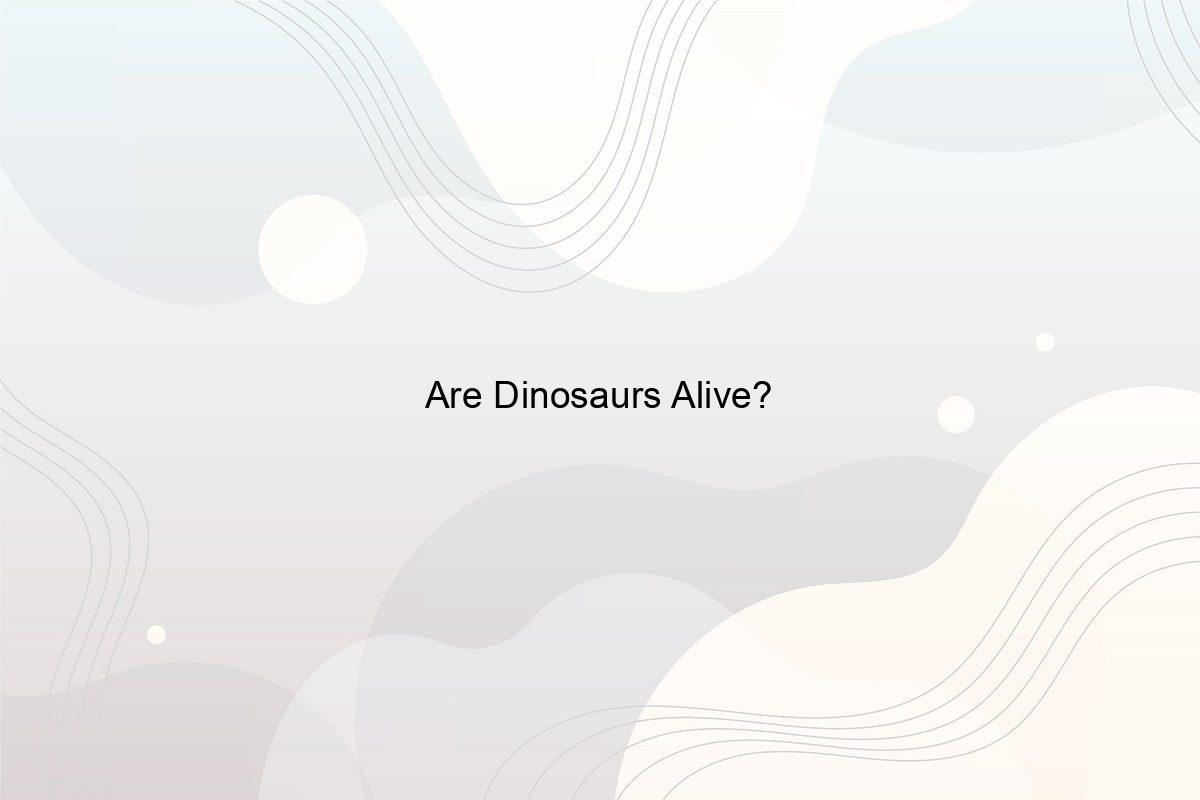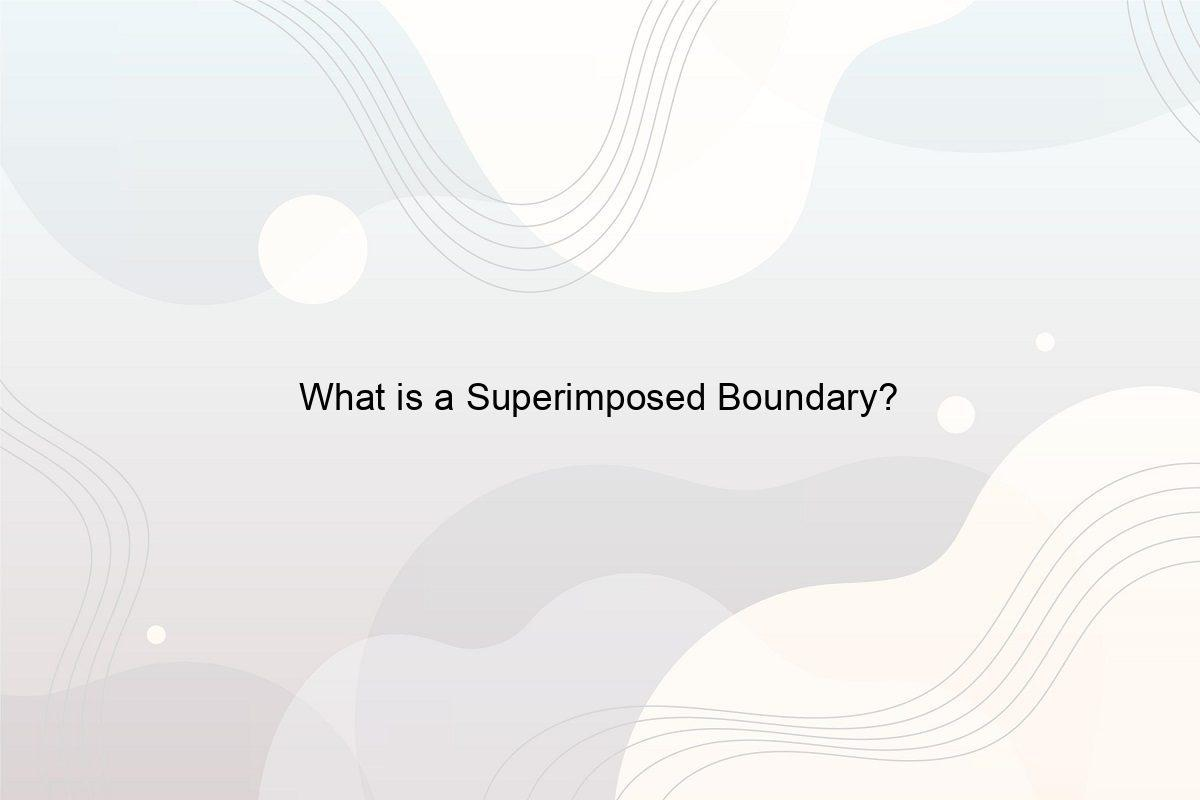﻿ What is the Very Last Number in the World? - Speeli

# What is the Very Last Number in the World?

Do Numbers end? What is the Last Number in the World? What is Vigintillion? What is Unvigintillion? What is Rayo’s number? What are Infinity and Eternity? Is Pi an infinite number?

Numbers are crucial to our existence. They constitute the soul of math and enable progress in science and technology. The concept of numbers is endless in theory. With the number line concept, which has integers, fractions, rational, and even irrational or imaginary numbers, you will never find yourself short of numbers. Despite an endless array of numbers, in theory, there is only so much we can practically count. So, you may ask what is the very last number in the world. In this article, you will learn what is the last number in the world, the concept of infinity, and a whole bunch about the types of  numbers.

### 1. Do Numbers end?

Image by Gerd Altmann from Pixabay

The common-sensical and short answer is no. However, instead of taking the answer at face value, let us attempt to understand the reason. The very conception of numbers gives it a never-ending status. Any number you think of, despite its magnitude, will always have a predecessor and a successor. However, when trespassing into the endpoints of numbers, you come across the concept of numbers being countably infinite. Numbers are countably infinite as you can count, in theory, to any given number. So, numbers do not end. (See When Were Numbers invented?)

### 2. What is the Very Last Number in the World?

It is not possible to answer what is the very last number in the world as the concept of numbers lacks a conclusive end. In theory, numbers can go on for as long as we can count. To understand these extremely large numbers, mathematicians and scientists use the concept of infinity. Infinity is not a number but rather a concept to denote unquantifiability. (See What are the Types of Functions?)

### 3. What is the Name of the Last Number?

As we established that numbers have no end, there is no number to call the last. However, there is the concept of infinity that answers this question to some effect. It is representative of numbers you cannot quantify, and you cannot quantify the last number either. (See What is a Circle Degree Chart?)

### 4. What is the Second Last Number in the World?

A logical step in answering what is the very last number in the world would be to first learn of its predecessor. However, numbers are such that they do not have a quantifiable end. Instead, infinity is the closest you have come to understand the end of numbers. Hence, there is no second last number in the world. (See How many cm in a Meter?)

### 5. Which is the Last Number in Maths?

What is the very last number in the world? There is none. In the world of mathematics in general, there is an infinite amount of numbers. In theory, there is no end to numbers. Hence, we cannot provide an answer for what is the very last number in the world. (See How to Write 1 Million in Numbers?)

### 6. What is the Number 1000000000000000000000000 called?

While anything over a billion seems to have one too many zeroes, the number above seems especially excessive. The number has 24 zeroes in case you lost count. You can express it mathematically as 10 to the power 24. However, if math is not for you, you can always refer to it by its name, septillion. Also, check out how long is 1 Billion Seconds in Hours?

### 7. What is Vigintillion?

Photo by Digital Buggu

A Vigintillion, like many others, means different things in the UK and US. A constant, however, is that a vigintillion is a very big number.

• In the US, a vigintillion is one followed by 63 zeroes or, mathematically, 10 to the power 63.
• However, in the UK, a vigintillion is one followed by 120 zeroes or, mathematically, 10 to the power 120. (See How long is 1 Million Hours?)

### 8. How many Zeros are in a Unvigintillion?

An unvigintillion is a number greater than a Vigintillion, in the US at least. An Unvigintillion is one followed by 66 zeroes. The mathematical expression for the same is 10 to the power 66.

### 9. What is a Quattuorvigintillion?

A Quattorviginitillion stands across a small jump from an Unvingitiliion. A Quattorvigintillion is a number greater than both a Vingitillion and an Unvingitillion. A Quattorvigintilion is one followed by 75 zeroes. The mathematical expression for the same is 10 to the power 75. (See What is Three Eighths as a Decimal?)

### 10. What is Rayo’s Number?

In trying to find what is the very last number in the world, we stumble across infinity. In our pursuit of infinity, you use finitely big numbers like googol or Rayo’s number to understand it. Rayo’s number is finitely big, a number series of exponentially large numbers. Rayo’s number in specific is the smallest number with less than 10 to the power 100 (or googol) symbols.

A Rayo’s number needs to be expressible in a set using first-order theory language. A set here refers to the mathematical function. The first-order theory language is a sequence of general statements from which you draw a logic. In case you were wondering if this is what is the last number in the world, you’re wrong. To know more about it, check out how many is a Googol?)

### 11. What is the Highest Number before Infinity?

This might seem to answer the question: what is the very last number in the world. However, infinity is not a number but rather a conceptual expression. It represents numbers you know exist but cannot quantify. As infinity is not a number and it lacks a predecessor. Therefore, no highest number comes before infinity. Must read what is Algebra used for in real life?

### 12. What is after Infinity?

Image by Gerd Altmann from Pixabay

Again, infinity is not a number. It would be more appropriate to call it an expression of numbers you cannot quantify. Therefore, no number is greater than infinity. The closest you can come to a successor is absolute infinity. Therefore, infinity plus one is essentially infinity. (See What Comes After a Trillion?)

### 13. Is Eternity bigger than Infinity?

Eternity and infinity, while seeming to be the same, are two different concepts. They share similarities in that they talk of quantifiability or a lack thereof. Eternity is a concept that theology explores in detail. It refers to time and something that has no beginning or end. (See Theory of Eternity of Life

On the other hand, infinity is a concept that science explores in theory. It refers to a value that you cannot quantify. Therefore, given the different nature of both these concepts, one cannot answer the question as to whether eternity is bigger than infinity. Also, check out how long is a decade and a century?

### 14. What is Infinity Minus Infinity?

Infinity minus infinity is a tricky one. Considering that integers exist, the answer to this question lies between negative infinity to 0 to positive infinity. Therefore, infinity minus infinity results in an undefined number

### 15. What is Infinity Plus Infinity?

Infinity is less of a quantity and more of a concept. Therefore, the sum of infinity and infinity results in a bigger infinity. Due to the lack of a distinct value, this larger infinity is just that, infinity. And there you have it, infinity plus infinity equals infinity. (See How many Sides does a Pentagon have?)

### 16. Why is Infinity Times Zero not Zero?

Throughout life, you learn that any number you multiply with zero results in a zero. Therefore, common sense dictates that infinity times zero will follow suit and result in a zero. However, in reality, infinity times zero is not zero. Infinity is not a number in the first place. When you multiply infinity with zero, you get an undefined number for a product. (Also read  Flip a Coin 100 Times How many Heads or Tails would appear?)

### 17. Is Pi Infinite?

Image by Gerd Altmann from Pixabay

Yes, as Johann Lambert proved to the world, Pi is infinite. However, this conclusion was a mere by-product. All irrational numbers are infinite, and that is what Mr. Lambert proved, that Pi is irrational. Infinity is complicated, and that is what you see here. Pi is infinite not because of its value but because of its expression. That is to say that the value of Pi is definite.

For example, Pi falls over three but under four. However, the expression of Pi is infinite. Pi is a non-recurring, non-terminating, and irrational number. As the Internet eloquently put it, Pi is like the trouble teenagers face, low lever but never-ending. To know more about it, check out What is pi? What is the meaning of Pi?

##### Related Posts## What Is a Pothole?

What pothole means? What is a Pothole? How are potholes formed?## Are Dinosaurs Alive?

Which was the Last Dinosaur seen alive? Which Dinosaur lived the Longest? Why & How did they become Extinct?